# Calculator sum of two mixed numbers

The calculator performs basic and advanced operations with mixed numbers, fractions, integers, decimals. Mixed fractions are also called mixed numbers. A mixed fraction is a whole number and a proper fraction combined, i.e. one and three-quarters. The calculator evaluates the expression or solves the equation with step-by-step calculation progress information. Solve problems with two or more mixed numbers fractions in one expression.

### 13/4 + 23/8 = 33/8 = 4 1/8 = 4.125

Spelled result in words is four and one eighth (or thirty-three eighths).

### Calculation steps

1. Conversion a mixed number 1 3/4 to a improper fraction: 1 3/4 = 1 3/4 = 1 · 4 + 3/4 = 4 + 3/4 = 7/4

To find a new numerator:
a) Multiply the whole number 1 by the denominator 4. Whole number 1 equally 1 * 4/4 = 4/4
b) Add the answer from previous step 4 to the numerator 3. New numerator is 4 + 3 = 7
c) Write a previous answer (new numerator 7) over the denominator 4.

One and three quarters is seven quarters
2. Conversion a mixed number 2 3/8 to a improper fraction: 2 3/8 = 2 3/8 = 2 · 8 + 3/8 = 16 + 3/8 = 19/8

To find a new numerator:
a) Multiply the whole number 2 by the denominator 8. Whole number 2 equally 2 * 8/8 = 16/8
b) Add the answer from previous step 16 to the numerator 3. New numerator is 16 + 3 = 19
c) Write a previous answer (new numerator 19) over the denominator 8.

Two and three eighths is nineteen eighths
3. Add: 7/4 + 19/8 = 7 · 2/4 · 2 + 19/8 = 14/8 + 19/8 = 14 + 19/8 = 33/8
For adding, subtracting, and comparing fractions, it is suitable to adjust both fractions to a common (equal, identical) denominator. The common denominator you can calculate as the least common multiple of both denominators - LCM(4, 8) = 8. In practice, it is enough to find the common denominator (not necessarily the lowest) by multiplying the denominators: 4 × 8 = 32. In the following intermediate step, the fraction result cannot be further simplified by canceling.
In other words - seven quarters plus nineteen eighths = thirty-three eighths.

## Mixed fractions in word problems:Add this two mixed numbers: 1 5/6 + 2 2/11=
• A more than bWhat is 5 1/7 more than 2 3/7?
• A science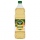A science experiment calls for making 3 and 2/3 cups of distilled water with 1 and 3/5 cups of vinegar and 2/3 cups of liquid detergent. How much liquid in all and cups is needed?Add this two mixed numbers: 1 5/6 + 2 2/11=Add two mixed fractions: 2 4/6 + 1 3/62 and 1/8th plus 1 and 1/3rd =
• Jack planted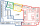Jack planted 1 1/4 rows of lettuce in the family garden, Rose planted 1 1/2 rows of broccoli and Calvin planted 4 5/12 rows of beans. How many rows were planted in the garden?
• Sum of mixedThe sum of two mixed numbers is 15½. One of the number is 5 ¾, what is the other?
• Dog food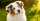A whole bag of dog food weighs 5 1/2 pounds. How much dog food do you have if there are 3 3/4 bags in the cupboard?
• Zailene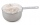Zailene wants to make two kinds of kakanin, puto and kutsina. Puto recipe needs 3 2/5 cup of flour while Kutsinta needs 5 ¼ cup of flour. How many cups of flour will Zailene be needed in all?3 3/4 + 2 3/5 + 5 1/2 Show your solution.The first team member in a 926-person relay race must run 2 1/4 laps, the second team member must run 1 1/2 laps, and the third team member must run 3 1/4 laps. How many laps in all must each team run?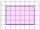You pick up donuts for a party. You have 3 and 4/12 boxes of donuts. Roy picks up donuts too. He has 2 and 9/12 boxes of donuts. How many boxes of donuts do you have in all?Sum of two fractions is 4 3/7. If one of the fractions is 2 1/5 find the other one .Sum of the two numbers is 15 1/6. If one of the numbers is 2 2/3, find the other number.Three scouts brought three pieces of rope of length five and three fourth meter, six and two third meters and five and five eights meters to their camps . how long are the 3 pieces together?Honey jogged 1 3/4 km on Monday,1 1/2 km on Wednesday and 1  2/3 km on Friday . how far did he jog?